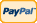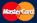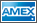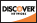Online Store Software Catalog and Price Product Registration Services News About Us Contact Us My Account Español
•Electronics, electrical engineering
•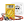Engineering physics
•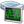Generators
•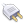Interfaces
•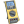Multimeters
•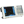Oscilloscopes
•Persona
•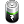Power supplies
•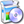Software
•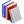Standards
•Thesaurus
•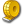Units of measurement
Filter by first letter
A
B
C
D
E
F
G
H
I
J
K
L
M
N
O
P
Q
R
S
T
U
V
W
X
Y
Z
+
-

# Design Engineer Valued Creative Encyclopedia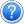Search: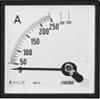The ampere (symbol: A) is the SI unit of electric current (symbol: I) and is one of the seven SI base units. It is named after André-Marie Ampère (1775–1836), French mathematician and physicist, considered the father of electrodynamics. In practice, its name is often shortened to amp.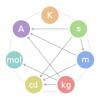Measurement unit that is adopted by convention for a base quantity. For example: The International System of Units (SI) defines seven units of measure as a basic set from which all other SI units are derived.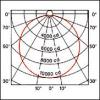The candela (symbol: cd) is the SI base unit of luminous intensity; that is, power emitted by a light source in a particular direction, weighted by the luminosity function (a standardized model of the sensitivity of the human eye to different wavelengths, also known as the luminous efficiency function).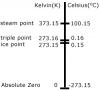Unit of measurement for temperature. It is named after the Swedish astronomer Anders Celsius, who developed a similar temperature scale.

SI derived unit of electric charge. It is defined as the charge transported by a steady current of one ampere in one second.Measurement unit for a derived quantity. Examples: the metre per second (m/s) and the centimeter per second (cm/s) are derived units of speed in the SI. The kilometre per hour (km/h) is a unit of speed outside the SI but accepted for use with the SI. The knot, equal to one nautical mile per hour, is a unit of speed outside the SI.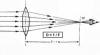A unit of measurement of the optical power of a lens or curved mirror, which is equal to the reciprocal of the focal length measured in metres (that is, 1/metres).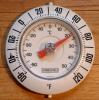Temperature scale proposed in 1724 by, and named after, the German physicist Daniel Gabriel Fahrenheit.

SI derived unit of capacitance named after English physicist Michael Faraday.

The SI derived unit of inductance.

The hertz (symbol Hz) is the SI unit of frequency defined as the number of cycles per second of a periodic phenomenon.

The joule, symbol J, is a derived unit of energy, work, or amount of heat in the International System of Units.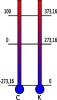The kelvin is a unit of measurement for temperature. It is one of the seven base units in the International System of Units (SI) and is assigned the unit symbol K.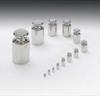The kilogram or kilogramme (kg) is the base unit of mass in the International System of Units (SI) which is the modern standard governing the metric system.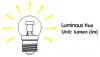The SI derived unit of luminous flux, a measure of the total "amount" of visible light emitted by a source.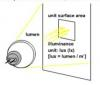The SI unit of illuminance and luminous emittance, measuring luminous flux per unit area.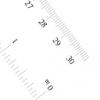It is a scalar quantity, defined and adopted by convention, with which any other quantity of the same kind can be compared to express the ratio of the two quantities as a number.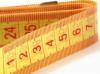The metre (or meter), symbol m, is the base unit of length in the International System of Units (SI).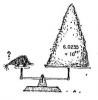The mole is a unit of measurement used in chemistry to express amounts of a chemical substance. It is one of the base units in the International System of Units, and has the unit symbol mol.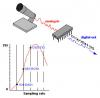MSa/s(MegaSAmples per Second) A measurement of sampling rate in millions of samples per second

GSa/s(GigaSAmples per Second) A measurement of sampling rate in giga of samples per second

SI derived unit of force. It is equal to the amount of net force required to accelerate a mass of one kilogram at a rate of one meter per second squared.

SI derived unit of pressure, internal pressure, stress, Young's modulus and tensile strength, named after Blaise Pascal.

The standard unit of angular measure, used in many areas of mathematics. An angle's measurement in radians is numerically equal to the length of a corresponding arc of a unit circle, so one radian is just under 57.3 degrees (when the arc length is equal to the radius).The second (SI symbol: s), sometimes abbreviated sec., is a unit of measurement of time, and is the International System of Units (SI) base unit of time. It may be measured using a clock.

The siemens (SI unit symbol: S) is the unit of electric conductance, electric susceptance and electric admittance in the International System of Units (SI).

The volt (symbol: V) is the SI derived unit for electric potential (voltage), electric potential difference, and electromotive force. The volt is named in honor of the Italian physicist Alessandro Volta.

The watt (symbol: W) is a derived unit of power in the International System of Units (SI), named after the Scottish engineer James Watt.

Articles 1 - 27 of 27
First | Prev. | 1 | Next | Last Paged
Units Converter
• How it Works
• Company News
• Industry News & Events
• Measurement Fun Facts